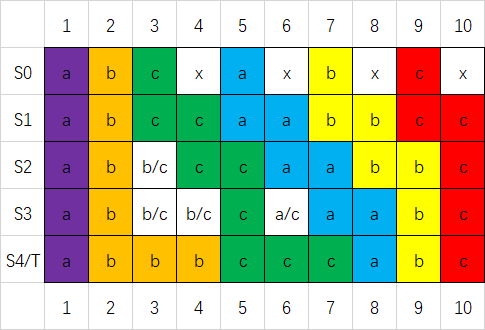AtCoder

## 题意简述

$$S_{i+1}[j]=\begin{cases}S_i&j=1\\S_i[j]\text{ 或 }S_{i+1}[j-1]&j>1\end{cases}$$

## 简要做法（如果您在色觉方面存在障碍，还请见谅。）

## 参考代码

#include <iostream>
#include <cstdio>
#include <string>
#include <queue>
#include <algorithm>

using namespace std;

int n, ans;
string s, t;
queue<int> q;

int main()
{
cin >> n >> s >> t;

if (s == t) // 特判两串相等
{
puts("0");
return 0;
}

int up = n - 1, down = n - 1;

while (down >= 0)
{
while (down && t[down - 1] == t[down]) --down; // 找到当前折线在最后一行最左的位置
while (up >= 0 && (up > down || s[up] != t[down])) --up; // 找到当前折线在第一行最左的位置
if (up < 0) // 如果第一行没有对应的字符，输出无解
{
puts("-1");
return 0;
}
while (!q.empty() && q.front() - q.size() >= down) q.pop(); // 把当前折线不会碰到的部分弹出
if (up != down) q.push(up); // 如果当前折线真的是“折线”而不是竖直下来不拐弯，就把 S1 的拐点压入队列
ans = max(ans, (int)q.size() + 1); // 后文会解释为什么这样更新答案
--down;
}

cout << ans;

return 0;
}


## 补充说明

• 对于最右的那条折线，显然成立。
• 对于之后的每条折线，一定是先贴着上一条折线，再直接往下到最后一行。由于上一条折线满足引理，如果中途有一段没有拐点而后又出现拐点，中途的那一段就没有紧贴上一条折线。